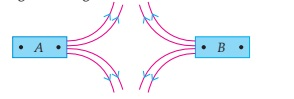Past Year - 5 Mark Questions

Class 10
Chapter 13 Class 10 - Magnetic Effects of Electric Current

## (a) Magnetic field lines of two bar magnets A and B are as shown below. Name the poles of the magnets facing each other.## (iii) Strength of the current flowing in the coil?

(a)

Magnetic field lines arise from the North pole and end into the South pole of the magnet.

In the given figure, magnetic field lines are arising from the poles facing each other . So, the poles of the magnet facing each other are north poles .

(b)

If two magnetic field lines intersect each other, then at that point, the magnetic field will have two directions , which is impossible . Hence, two magnetic field lines never intersect each other.

(c)

The strength of the magnetic field at the center of a current carrying circular coil is:

1. Inversely proportional to the radius of the coil : If the radius increases, the strength of the magnetic field decreases.
2. Directly proportional to the number of turns in the coil: If the number of turns increases, the strength of the magnetic field increases.
3. Directly proportional to the strength of the current flowing in the coil: If the strength of current increases, the strength of the magnetic field increases.

Get live Maths 1-on-1 Classs - Class 6 to 12